# Similar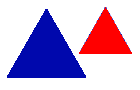Two shapes are Similar when the only difference is size (and possibly the need to turn or flip one around).

## Resizing is the Key

If one shape can become another using Resizing (also called dilation, contraction, compression, enlargement or even expansion), then the shapes are Similar:

 These Shapes are Similar!## There may be Turns, Flips or Slides, Too!

Sometimes it can be hard to see if two shapes are Similar, because you may need to turn, flip or slide one shape as well as resizing it.

 Rotation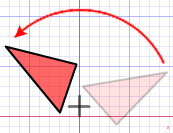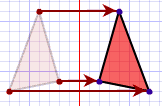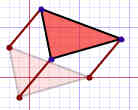## Examples

These pairs of shapes are all Similar: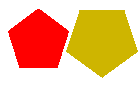Resized Resized and Reflected Resized and Rotated

## Why is it Useful?

When two shapes are similar, then:

• corresponding angles are equal, and
• the lines are in proportion.

This can make life a lot easier when solving geometry puzzles, as in this example:

### Example: What is the missing length here?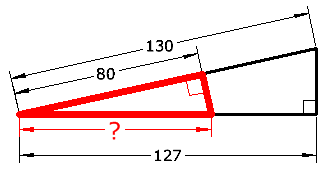Notice that the red triangle has the same angles as the main triangle ...

... they both have one right angle, and a shared angle in the left corner

In fact you could flip the red triangle over, rotate it a little, then resize it and it would fit exactly on top of the main triangle. So they are similar triangles.

So the line lengths will be in proportion, and we can calculate:

### ?= 80 × (130/127) = 81,9

(No fancy calculations, just common sense!)

## Congruent or Similar?

Shapes are Congruent when they are the same size (but may have been rotated, reflected or moved). So when the shapes become the same:

When we ...   Then the shapes are ...
... only Rotate, Reflect and/or Translate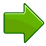### Congruent

... also need to Resize## Are Congruent Shapes also Similar?

Most people (including us) say "Congruent shapes are also Similar".

### Example:We can move and rotate the orange shape to exactly match the blue shape, so the two shapes are Congruent.

We don't have to resize for the shapes to be similar! So they are also Similar even though no resizing was needed.